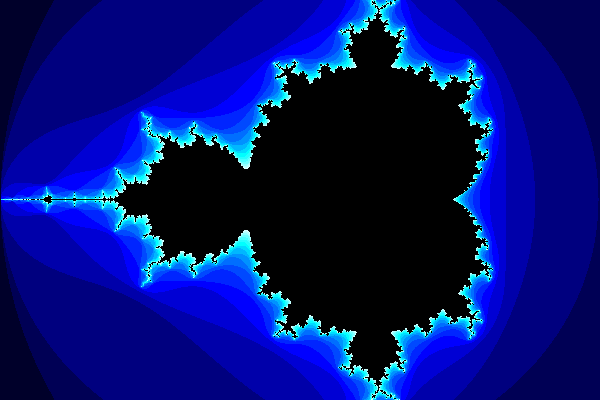exupero's blog

# Drawing the Mandelbrot set

For all its infinite complexity, I was surprised to learn how simple it is to generate the Mandelbrot set. All it requires is complex addition, multiplication, distance, and some iteration. Here's a worked example.

We'll represent complex numbers as two-element vectors, where the first element is the real part and the second is the imaginary part. Complex addition is simply componentwise addition:

``````(defn complex-add [a b]
(mapv + a b))``````

Complex multiplication is more elaborate, but not difficult:

``````(defn complex-multiply [[r1 i1] [r2 i2]]
[(- (* r1 r2) (* i1 i2))
(+ (* r1 i2) (* r2 i1))])``````

The distance from a point in the complex plane to the origin is regular Euclidean distance:

``````(defn distance-to-origin [[r i]]
(Math/sqrt (+ (* r r) (* i i))))``````

Now for the hard work. The mathematical definition of the Mandelbrot set is f(z) = z2 + c, but that omits a lot of the actual algorithm. To create the traditional image of the Mandelbrot set, we need to call that function over and over and see how quickly the result diverges from its starting point:

``````(defn mandelbrot [c]
(loop [i 0
z [0 0]]
(if (and (< i 20)
(< (distance-to-origin z) 2))
(recur (inc i) (complex-add c (complex-multiply z z)))
i)))``````

The above function loops up to 20 times, or quits as soon as the point moves more than two units from its starting point. To draw the set, we'll color points in an image based on how quickly they diverged past a two-unit radius. Here's a list of hexadecimal RGB colors that walk up through blues, then add increasing amounts of green, then red:

``````(def color
[nil
0x00002a
0x000055
0x00007f
0x0000aa
0x0000d4
0x0000ff
0x0025ff
0x0049ff
0x006eff
0x0092ff
0x00b7ff
0x00dbff
0x00ffff
0x25ffff
0x49ffff
0x6effff
0x92ffff
0xb7ffff
0xdbffff
0x000000])``````

Mapping each pixel in an image to the complex plane with corners -2 - i and 1 + i produces this:While the bright, complex edge is what usually draws our attention in images like these, the colored points above don't actually belong to the Mandelbrot set. Only those points that don't diverge to infinity when iterated through f(z) = z2 + c are part of the set; that is, the points shown in black. The Mandelbrot set is usually illustrated as negative space.